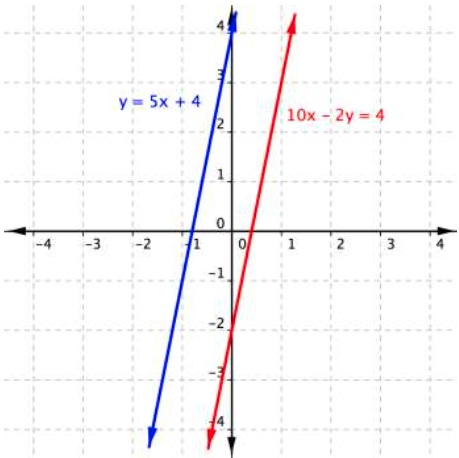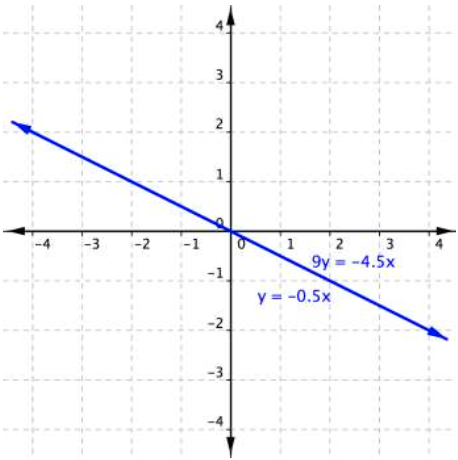## The Substitution Method

### Learning Outcomes

• Use the substitution method to solve systems of equations
• Express the solution of an inconsistent system of equations containing two variables
• Express the solution of a dependent system of equations containing two variables

## Solve a system of equations using the substitution method

In the last couple sections, we verified that ordered pairs were solutions to systems, and we used graphs to classify how many solutions a system of two linear equations had. Solving a linear system in two variables by graphing works well when the solution consists of integer values, but if our solution contains decimals or fractions, it is not the most precise method.  What if we are not given a point of intersection, or it is not obvious from a graph? Can we still find a solution to the system? Of course you can, using algebra!

In this section we will learn the substitution method for finding a solution to a system of linear equations in two variables. We have used substitution in different ways throughout this course. For example, when we were using the formulas for the area of a triangle and simple interest we substituted values that we knew into the formula to solve for values that we did not know.  The idea is similar when applied to solving systems, there are just a few different steps in the process. In the substitution method we solve one of the equations for one variable and then substitute the result into the other equation to solve for the second variable.  Recall that we can solve for only one variable at a time which is the reason the substitution method is both valuable and practical. Let’s start with an example to see what this means.

### Example

Find the value of $x$ for this system.

Equation A: $4x+3y=−14$

Equation B: $y=2$

You can substitute a value for a variable even if it is an expression. Here’s an example.

### Example

Solve for $x$ and $y$.

Equation A: $y+x=3$

Equation B: $x=y+5$

Remember, a solution to a system of equations must be a solution to each of the equations within the system. The ordered pair $(4,−1)$ does work for both equations, so you know that it is a solution to the system as well.

In the examples above, one of the equations was already given to us in terms of the variable $x$ or $y$. This allowed us to quickly substitute that value into the other equation and solve for one of the unknowns.

Sometimes you may have to rewrite one of the equations in terms of one of the variables first before you can substitute. In the example below, you will first need to isolate one of the variables before you can substitute it into the other equation.

### Example

Solve the following system of equations by substitution.

$\begin{array}{l}-x+y=-5\hfill \\ \text{ }2x - 5y=1\hfill \end{array}$

Here is a summary of the steps we use to solve systems of equations using the substitution method.

### How To: Given a system of two equations in two variables, solve using the substitution method

1. Solve one of the two equations for one of the variables in terms of the other.
2. Substitute the expression for this variable into the second equation, and then solve for the remaining variable.
3. Substitute that solution into either of the original equations to find the value of the other variable. If possible, write the solution as an ordered pair.
4. Check the solution in both equations.

Let’s look at some examples whose substitution involves the distributive property.

### Example

Solve for $x$ and $y$.

$\begin{array}{l}y = 3x + 6\\−2x + 4y = 4\end{array}$

### Example

Solve for $x$ and $y$.

$\begin{array}{r}2x+3y=22\\3x+y=19\end{array}$

In the following video, you will be given an example of solving a systems of two equations using the substitution method.

If you had chosen the other equation to start with in the previous examples, you would still be able to find the same solution.  It is really a matter of preference because sometimes solving for a variable will result in having to work with fractions.  As you become more experienced with algebra, you will be able to anticipate what choices will lead to more desirable outcomes.

## Identify systems of equations that have no solution or an infinite number of solutions

Recall that an inconsistent system consists of parallel lines that have the same slope but different y-intercepts. They will never intersect. When searching for a solution to an inconsistent system, we will come up with a false statement such as $12=0$.

When we learned methods for solving linear equations in one variable, we found that some equations didn’t have any solutions, and others had an infinite number of solutions.  We saw this behavior again when we started describing solutions to systems of equations in two variables.

Recall this example from Module 1 for solving linear equations in one variable:

Solve for $x$. $12+2x–8=7x+5–5x$

$\displaystyle \begin{array}{l}12+2x-8=7x+5-5x\\\,\,\,\,\,\,\,\,\,\,\,\,\,\,2x+4=2x+5\end{array}$

$\begin{array}{l}\,\,\,\,\,\,\,\,\,\,\,\,2x+4=2x+5\\\,\,\,\,\,\,\,\,\underline{-2x\,\,\,\,\,\,\,\,\,\,-2x\,\,\,\,\,\,\,\,}\\\,\,\,\,\,\,\,\,\,\,\,\,\,\,\,\,\,\,\,\,\,\,\,\,4= \,5\end{array}$

This false statement implies there are no solutions to this equation.  In the same way, you may see an outcome like this when you use the substitution method to find a solution to a system of linear equations in two variables. In the next example, you will see an example of a system of two equations that does not have a solution.

### Example

Solve for $x$ and $y$.

$\begin{array}{l}y=5x+4\\10x−2y=4\end{array}$

You get the false statement $−8=4$. What does this mean? The graph of this system sheds some light on what is happening.The lines are parallel, they never intersect and there is no solution to this system of linear equations. Note that the result $−8=4$ is not a solution. It is simply a false statement and it indicates that there is no solution.

Let’s look at another example in which there is no solution.

### Example

Solve the following system of equations.

$\begin{array}{l}x=9 - 2y\hfill \\ x+2y=13\hfill \end{array}$

In the next video, we show another example of using substitution to solve a system that has no solution.

### Try It

We have also seen linear equations in one variable and systems of equations in two variables that have an infinite number of solutions.  In the next example, you will see what happens when you apply the substitution method to a system with an infinite number of solutions.

### Example

Solve for $x$ and $y$.

$\begin{array}{l}\,\,\,y=−0.5x\\9y=−4.5x\end{array}$

This time you get a true statement: $−4.5x=−4.5x$. But what does this type of answer mean? Again, graphing can help you make sense of this system.This system consists of two equations that both represent the same line; the two lines are collinear. Every point along the line will be a solution to the system, and that’s why the substitution method yields a true statement. In this case, there are an infinite number of solutions.

### Try It

In the following video you will see an example of solving a system that has an infinite number of solutions.

In the following video you will see an example of solving a system of equations that has no solutions.

## Summary

The substitution method is one way of solving systems of equations. To use the substitution method, use one equation to find an expression for one of the variables in terms of the other variable. Then substitute that expression in place of that variable in the second equation. You can then solve this equation as it will now have only one variable. Solving using the substitution method will yield one of three results: a single value for each variable within the system (indicating one solution), an untrue statement (indicating no solutions), or a true statement (indicating an infinite number of solutions).

## Contribute!

Did you have an idea for improving this content? We’d love your input.